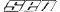Staredit Network > Forums > Lite Discussion > Topic: Integrate this!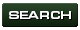Integrate this!
Oct 14 2010, 1:05 am
By: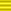Ahli

Oct 14 2010, 1:05 amAhli Post #1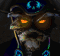I do stuff and thingies... Try widening and reducing the number of small nooks and crannies to correct the problem.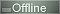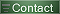My ranting/story/introduction:
Because of my school following the advice of my county's educational department, I am unable to integrate light complex formulas.
I've only really learned it how to solve everything with my programmable calculator (ti voyage 200) instead of calculating everything with my brain.
For my current course (statistics) at university I need to know how to integrate a few things.

Integral of x * sqrt( 1 - x ), dx from 0 to 1

My try:
So I've tried the integration per substitution with the help of the English wikipedia and other pages.
I've chosen:
u = 1 - u <=> x = 1 - u
du/dx = -1 <=> du = -dx

Now I see that I need a "-dx" in my formula to be able to substitute. So I add *(-1) twice (Correct?):
= integral( x * sqrt(1-x) (-1) (-1) dx) from 0 to 1

= integral( (1-u) * sqrt(u) * (-1) * du) from 0 to 1

= integral( - sqrt(u) + u * sqrt(u) * du) from 0 to 1

= integral( - sqrt(u) du)|0,1 + integral( u^(3/2) du)|0,1

= [-2/3 * u^(3/2)]|0,1 + [2/5 * u^(5/2)]|0,1

= [-2/3 * (1 - x)^(3/2)]|0,1 + [2/5 * (1 - x)^(5/2)]|0,1

= 2/3 + 2/5 = 16/15

But the result should be 4/15 (my calc says that & with integral from wolfram integral page I get the same result) (which would be 2/3 - 2/5).

What am I doing wrong and am I executing it correctly in general or am I am missing some thoughts?

Post has been edited 1 time(s), last time on Oct 14 2010, 1:26 am by Ahli. Reason: corrected "|1,0" -> "|0,1"Oct 14 2010, 1:30 am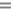Vrael Post #2First you need to learn how to make what is called an "Indefinite Integral."

A Definite Integral has an upper and lower bound of integration, for example, 0 to 1 of x*sqrt( 1 - x ) would be a definite integral, because you are integrating over the interval (0,1).

An Indefinite Integral is similar to the Definite Integral, except there are no bounds of integration. So instead of a number as the answer, we get a function as our answer.
The indefinite integral of a function f(x)dx = F(x). The definite integral is related to the indefinite integral. Essentially, if you evaluate the indefinite integral at the two endpoints of an interval, you get the definite integral. The integral from a to b of f(x)dx = F(a) - F(b), the indefinite integral evaluated at a minus the indefinite integral evaluated at b.

Another word for an indefinite integral is antiderivative. What I advise, is that you learn how to find antiderivatives, because once you can find them, you can plug in values at the end points and find the value of definite integrals.

Edit: I didn't read your whole post at the beginning lol. I thought you were asking for basic integration help.

If
u = 1 - x <=> x = 1-u
du = -dx <=> (-1)du = dx
x*sqrt(1-x) = (1-u)sqrt(u)(-1)du
Distributing the -1: (u - 1)sqrt(u) = u^3/2 - u^1/2
= (2/5)u^(5/2) - (2/3)u^(3/2)
antiderivative = (2/5)(1-x)^(5/2) - (2/3)(1 - x)^(3/2)
F(1) - F(0) = [ 0 ] - [ (2/5) - (2/3) ]
= 2/3 - 2/5 = 4/15

Post has been edited 3 time(s), last time on Oct 14 2010, 1:55 am by Vrael.

None.

Oct 14 2010, 8:49 amAhli Post #3I do stuff and thingies... Try widening and reducing the number of small nooks and crannies to correct the problem.Quote from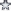Vrael
If
u = 1 - x <=> x = 1-u
du = -dx <=> (-1)du = dx
x*sqrt(1-x) = (1-u)sqrt(u)(-1)du
Distributing the -1: (u - 1)sqrt(u) = u^3/2 - u^1/2
= (2/5)u^(5/2) - (2/3)u^(3/2)
antiderivative = (2/5)(1-x)^(5/2) - (2/3)(1 - x)^(3/2)
F(1) - F(0) = [ 0 ] - [ (2/5) - (2/3) ]
= 2/3 - 2/5 = 4/15
thx, just learned that I just should solve the equation with dx and du to have dx alone and just replace it.
I don't know where I messed it up at start because that should have worked, too, but I'm doing it again with that knowledge and I'm getting the right result now.Oct 14 2010, 8:51 am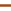Neki Post #4Told you, integration by substitution! Too bad I'm well past most of the Calculus I'll ever do in my lifetime, tis a shame because it's much easier than anything else I'm taking! I was going to solve it but then Vrael just confused me before he edited a solution in

None.

Oct 14 2010, 4:03 pmCecilSunkure Post #5The substitution you are referring to, Ahli, is u (yoo; yew; yu; you) substitution. Once again Vrael beats me to solving the math problem, so I suppose I don't need to solve it for you either.

None.

Oct 15 2010, 12:07 amVrael Post #6Quote from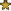Ahli
Quote fromVrael
If
u = 1 - x <=> x = 1-u
du = -dx <=> (-1)du = dx
x*sqrt(1-x) = (1-u)sqrt(u)(-1)du
Distributing the -1: (u - 1)sqrt(u) = u^3/2 - u^1/2
= (2/5)u^(5/2) - (2/3)u^(3/2)
antiderivative = (2/5)(1-x)^(5/2) - (2/3)(1 - x)^(3/2)
F(1) - F(0) = [ 0 ] - [ (2/5) - (2/3) ]
= 2/3 - 2/5 = 4/15
thx, just learned that I just should solve the equation with dx and du to have dx alone and just replace it.
I don't know where I messed it up at start because that should have worked, too, but I'm doing it again with that knowledge and I'm getting the right result now.
The idea is that the two expressions are exactly equivelent, so that when you replace all the x's with a u-expression-counterpart, you haven't changed the equation. The big problem sometimes with the "du" part, is that sometimes du != dx.
If say, u = 1- 3x^2, then du = -6x*dx since d(u) = d(1 - 3x^2) = -6xdx
So if you wanted to integrate something like x*ln(1 - 3x^2) dx, the equivelent expression would be (-1/6)*ln(u)*du
The (-1/6) "cancels out" with the -6 in the du expression, leaving just "x*dx" times the ln(1-3x^2) like we wanted.

I can elaborate more on what "dx" is if you need, I get the feeling a lot of people don't understand why its in the integral expression.

None.

Oct 16 2010, 4:53 pmAhli Post #7I do stuff and thingies... Try widening and reducing the number of small nooks and crannies to correct the problem.Thanks guys, I've managed to integrate everything correctly.

And yes, I don't know why the dx is in there. I know that df(x)/dx is the derivative of the function with x (after x, I don't know which words I have to use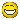).Oct 16 2010, 8:46 pmVrael Post #8An integral is the area beneath the curve, f(x). In calculus, you learn the idea of a limit, and an integral is the limit of a sum of terms. In the picture above, there are only 8 sub divisions beneath f(x). So if we multiply f(x) * dx 8 times, we'll get an area thats pretty close to the area beneath that curve, but it won't be exact. The more subdivisions we have, the closer the area will be to exactly the area beneath the curve. Without "dx" we wouldn't be calculating areas, because we wouldn't be summing areas, we'd be summing lengths. The idea of an integral is that dx is extremely small, so small in fact that there are infinitely many subdivisions, so when we calculate the area we get the exact area. Using limits, we can write
"The limit as dx approaches 0, of the sum of all rectangles f(x)*dx, over the interval (a,b)" = the integral from a to b of f(x).

In integrals, you can have a "negative" area. Thats how integrals like, from -1 to 1 of x^3 = 0. You have a negative area cancel out with a positive area. Also, it's been proven that the area beneath f(x) over the interval (a,b) = F(b) - F(a). That's the "fundamental theorem of calculus", where F(x) = antiderivative of f(x).

None.

Options
Back to forum
Members in this topic: None.
[05:00 pm]KrayZee -- Ultraviolet
Ultraviolet shouted: KrayZee Seems like you already know the answer. Don't feed the trolls
Funny that you write that. Someone just told me the exact same thing but about another person in that same forum who is acting pretty dumb.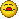[04:32 pm]Ultraviolet -- KrayZee
KrayZee shouted: How does one deal with a narcissist who is making things up, exaggerates their statements using words like "worst experience in my life" as to put emphasis in declaring trivial things, can't admit they are wrong and likes to escalate a situation that wasn't even a problem in the first place? I'm already at the point of just straight up ignoring this person and reporting em to forum moderators.
Seems like you already know the answer. Don't feed the trolls
[04:20 pm]NudeRaider -- Suicidal Insanity
Suicidal Insanity shouted: There was a blue post to that effect, why would it be silly?
so it is true, but still silly
[04:20 pm]NudeRaider -- Suicidal Insanity
Suicidal Insanity shouted: There was a blue post to that effect, why would it be silly?
not sure if there's a misunderstanding, because obviously blue posts can be silly as well.
[04:18 pm]NudeRaider -- Suicidal Insanity
Suicidal Insanity shouted: Sounds like trump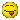because he's a narcissist as well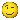[03:35 pm]Magicide -- feels good
[03:30 pm]Magicide -- tfw you log in after ages and check out the grps, and people have been using your muzzle flash sprites XD
[02:18 pm]
Suicidal Insanity -- Sounds like trump[01:43 pm]
MTiger156 -- ... wut?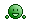[01:02 pm]KrayZee --Members Online:Roy,KrayZee, Hunsbergeraq70